Mr. Nolde's Polyhedron Origami Photo Gallery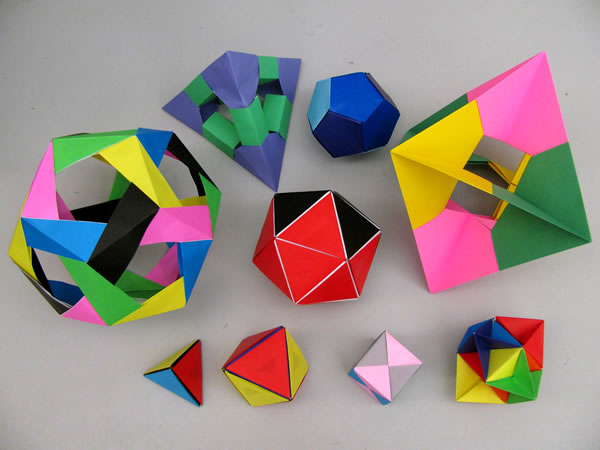"What is a polyhedron?" you ask.  Let's first review what a polygon is first!  A polygon is a 2-dimensional structure with some number of connected straight lines (edges) and corners (vertices).  Some polygons we call "regular polygons."  We call them regular polygons when all of their edges have the same length and the angle of all of their vertices are also all equal to one another.

A polyhedron is a 3-dimensional solid with polygonal faces.  The names of these shapes come from the Greek & Latin words.  For example, "tetra" means four, "hexa" is six, "octa" is eight, "dodeca" is twelve, and "icosa" is twenty.  Every polyhedron has vertices, edges, and faces enclosing a space: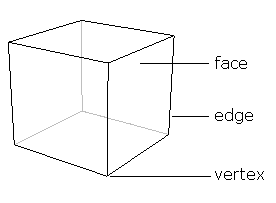A regular polyhedron is a polyhedron whose faces are all identical regular polygons and their vertices are all formed by the same number of faces.  For example, in our hexahedra (cube), we always have 3 faces forming a vertex. (See diagram above.)  If you look carefully, you will also see that the hexahedra has 6 identical faces that are all squares.  We of course, know that a square is a good example of a regular polygon.  In the figures below, see if you can figure out what type of identical regular polygon each of the following polyhedra has: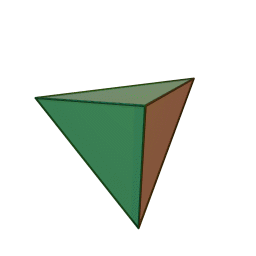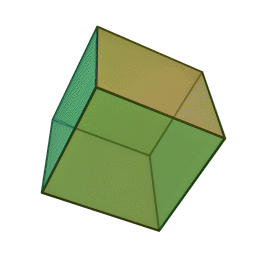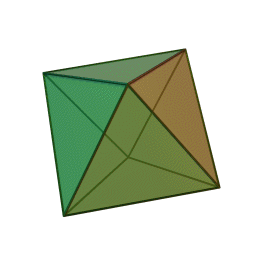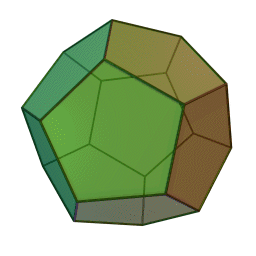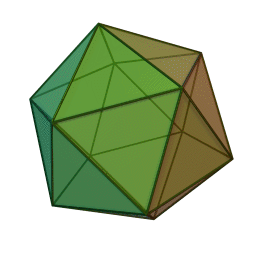Tetrahedron 4 Congruent Faces Hexahedron 6 Congruent Faces Octahedron 8 Congruent Faces Dodecahedron 12 Congruent Faces Icosahedron 20 Congruent Faces

Shown below, are photos of some of my origami.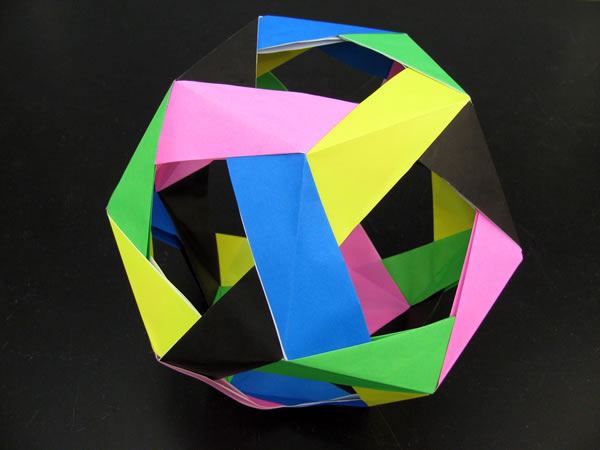Penultimate Dodecahedron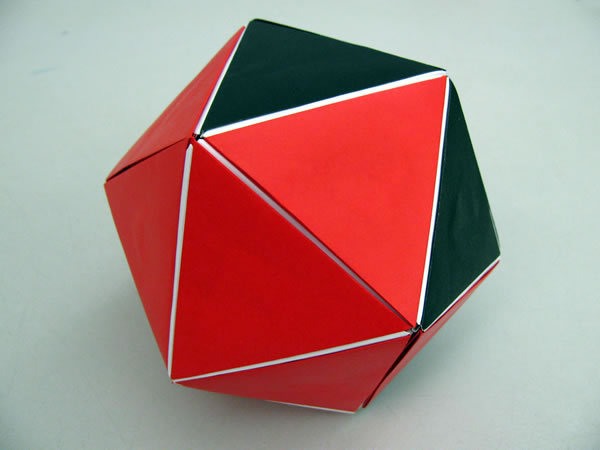Icosahedron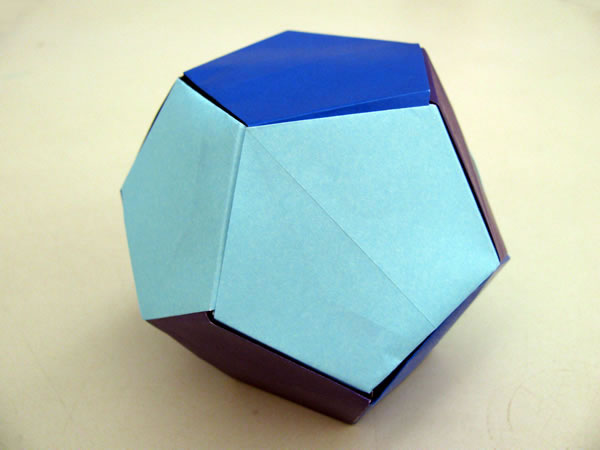Dodecahedron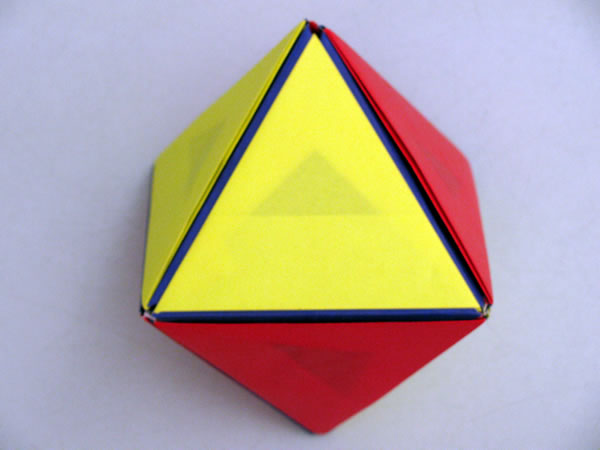Octahedron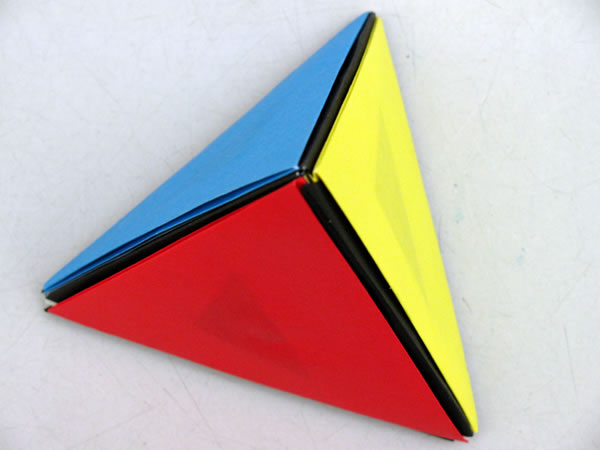Tetrahedron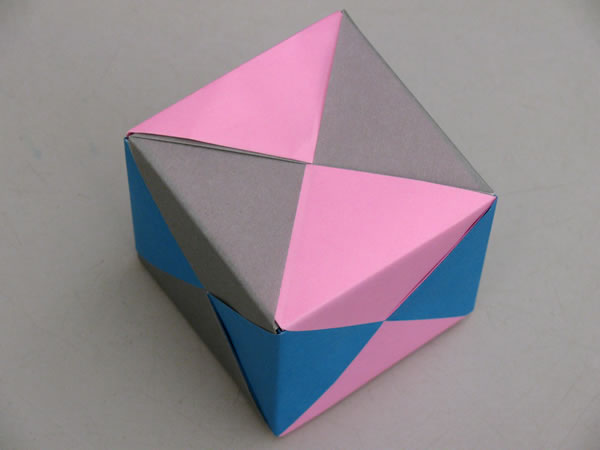Hexahedron (cube)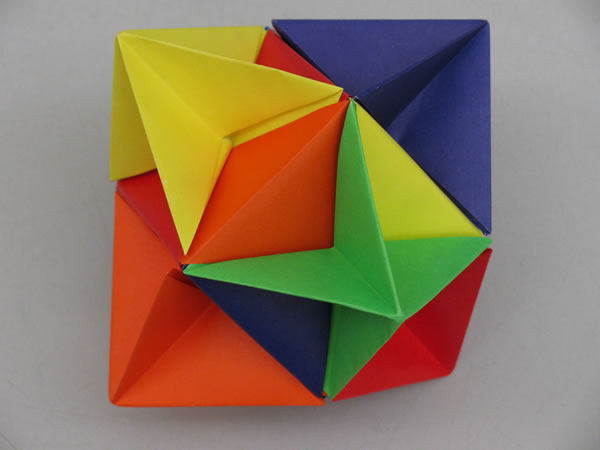Stellated Cube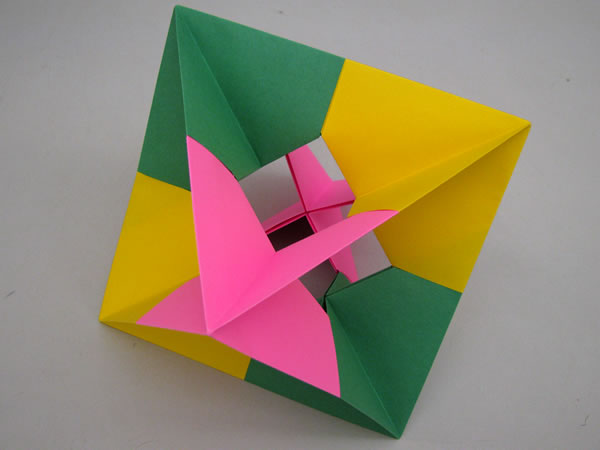Octahedral Skeleton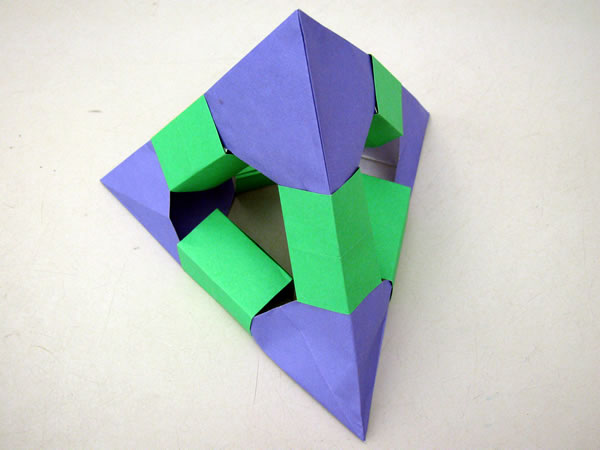Tetrahedron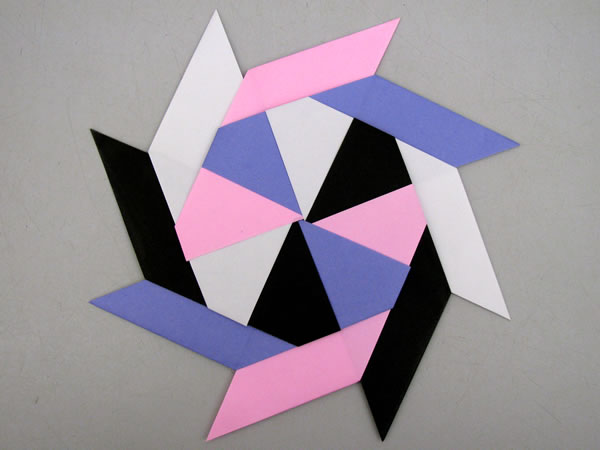The Ninja Star
(Not a polyhedron, but cool nonetheless!)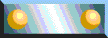Math Resource PageReturn to homepage